Mathematical and Physical Journal
for High Schools
Issued by the MATFUND Foundation
 Already signed up? New to KöMaL?

# KöMaL Problems in Physics, February 2011

Show/hide problems of signs:## Problems with sign 'M'

Deadline expired on March 10, 2011.

M. 312. Measure the speed of ripples of certain frequency on the surface of water. How does this speed depends on the depth of the water?

(6 pont)

statistics## Problems with sign 'P'

Deadline expired on March 10, 2011.

P. 4315. Two balls of masses m1=0.173 kg and m2=0.2 kg are moving and the direction of their motion is perpendiculer to each other. Their speeds are v1=10 m/s and v2=5 m/s, respectively. After the collision the ball of mass m1 stops. What will the speed of the ball of mass m2 be after the collision?

(4 pont)

solution (in Hungarian), statistics

P. 4316. Two filament bulbs rated at 6 V; 0.5 A and at 4 V; 0.4 A are to be operated from a 12 V rechargeable battery. The bulbs should be operating at their ratings. Plan a possible circuit using the appropriate resistors. How much energy is lost?

(4 pont)

solution (in Hungarian), statistics

P. 4317. A skewer of mass m=1 g is hanged with a short thread attached to a fixed point P. A long thread is attached to the bottom end of the skewer, and it is kept in equilibrium with a horizontal force. In this case the angle between the skewer and the vertical is. a) Find the angle between the short thread and the vertical. b) Find the tension in the short thread, if=45o.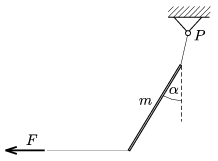(4 pont)

solution (in Hungarian), statistics

P. 4318. Determine with the help of a computer (with numerical method) how much shorter does it take for an iron ball of diameter 10 cm to fall from the balkony of the leaning tower of Pisa, which is at a height of 50 m, than a wooden ball of the same diameter.

(5 pont)

solution (in Hungarian), statistics

P. 4319. In a soap film experiment an originally semicircle shaped thread of length L can be deformed to two semicircles with a force of F exerted at the middle of the thread. Calculate the surface tension.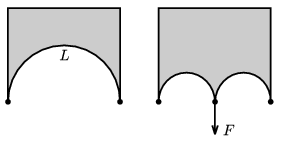(4 pont)

solution (in Hungarian), statistics

P. 4320. A lead bal of mass M hangs on a thread of length L. A lead bullet of mass m, and of horizontal velocity v hits the ball exactly at its centre. The bullet penetrates into the ball. Initially their temperature is the same. a) What is the greatest angle of deflection of the thread? b) At most how many degrees does the temperature of the lead increases? The specific heat capacity of lead is c.

(4 pont)

solution (in Hungarian), statistics

P. 4321. The thermal mean speed of neutral elementary particles is 2.7 km/s at a temperature of 22 oC. What is the average speed of these particles in the reactor container of a nuclear power station, where the temperature is 315 oC? What can these particles be?

(4 pont)

solution (in Hungarian), statistics

P. 4322. Subscribers can reach the text of the problem after signing in. The text will be public from February 12, 2011.]

(4 pont)

solution (in Hungarian), statistics

P. 4323. The wire shown in the figure contains a U-shaped part, and is placed to the metal stands such that it can turn easily. Each part of the U-shaped part has a length of=10 cm, and a mass of m=0.15 g.The wire is raised to horizontal position, and a long straight wire, which is parallel to the middle part of the U, is placed below it at a distance of h=2 mm. The two wires are connected in series to a current source. What should the current in the wire be, in order to keep the displaced U-shaped part in the horizontal when it is released?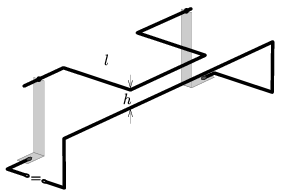(4 pont)

solution (in Hungarian), statistics

P. 4324. One end of a thread of length l=1 m is attached to an inclined plane of angle of elevation of=30o. A point-like body of mass m=1 kg is attached to the other end of the thread as shown in the figure. The body is released without initial speed, such that the thread is tight and horizontal. The coefficient of friction between the slope and the body is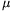=0.2. a) Determine the maximum speed of the sliding body. b) Find the tension in the thread when the angle between the thread and the horizontal is first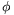=60o. c) In which position of the thread will the tension be maximum?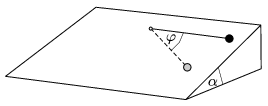(5 pont)

solution (in Hungarian), statistics

P. 4325. A three end thoroid, containing an iron core, is connected into a circuit as shown in the figure. What will the current through switch K be right after closing the switch, if the initial current in the circuit was I0? (The ohmic resistance of the coil is negligible with respect to R.)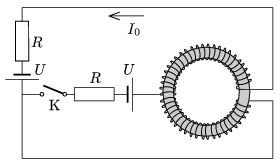(6 pont)

solution (in Hungarian), statistics